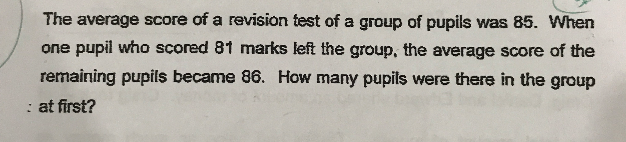QuestionSource: Anglo-Chinese School Primary

Number of pupils -> u

Total score of u pupils -> 85u

Number of pupils after 1 left -> (u – 1)

Total score of (u -1) pupils -> 85u – 81

Total score of (u -1) pupils is also -> new average × new number of pupils = 86 ×  (u – 1) = 86u – 86

85u – 81 = 86u – 86

u = 5

Number of pupils at first -> 5

0 Replies 1 Like ✔Accepted Answer

Method 1 :
86 – 81 = 5
86 – 85 = 1
5/1 = 5

Method 2 :
number of pupils x 85 ——- (number of pupils – 1) x 86 + 81
number of pupils ——- (86 – 81)(86 – 85) = 5

Ans : 5 pupils.

0 Replies 1 Like

Concept of sharing :

Let’s change the question a little bit.  Consider a group of children with an average of 85 sweets per child.  Then, one child left with 81 sweets. After that, the average number sweets each child had became 86.

When one child left with 81 sweets,  (85-81) = 4 sweets was left behind (since each had an average of 85 sweets at first).  So these 4 sweets can be shared with the remaining children.  Since each of the remaining children get (86-85) =  1 extra sweet each,

number of remaining children = 4 ÷ 1 = 4

Total number of children at first = 4+ 1 = 5.

cheers.

0 Replies 2 Likes